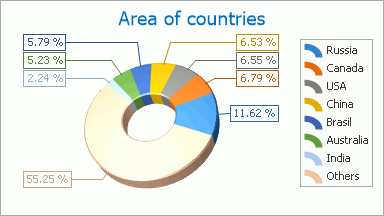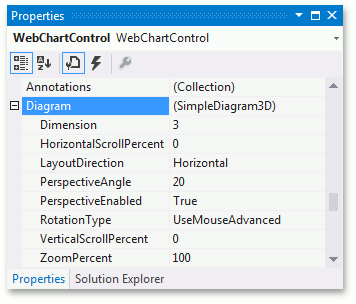# Simple Diagram 3D

This topic lists the series view types associated with the ASP.NET Chart Control’s Simple diagram 3D type. It also explains how to access specific options (both at design time and runtime), and briefly describes these options. Before reading this section, you may wish to review the following help topic on diagram fundamentals: Diagram.

The topic consists of the following sections.

## Associated Series View Types

You can use the WebChartControl to create attractive, high-resolution 3D versions of many of our 2D Chart Types. For example, the Simple diagram 3D type allows you to plot series of the Pie 3D and Doughnut 3D view types. A typical Simple 3D diagram is shown in the following image.The Pie and Doughnut series are useful when it is necessary to compare the percentage values of different point arguments in the same series. The points of a series are distributed along the circle’s radius, which is considered to be 100%. Each slice of this circle is a portion of the whole series. The size of a slice depends on a point’s value.

The only difference between the Pie and Doughnut series view types is visual: the Doughnut series has a hole in its center.

For the 3D diagram types a completely different graphical engine is used, to meet their specific requirements. For this reason, the 3D diagram has functionality that is unique to its type (in comparison to the 2D diagram type). Likewise, it’s impossible to plot 2D and 3D series within the same diagram.

For detailed descriptions of the options available for this diagram type, refer to the following section of this topic: Specific Options.

## Accessing a Diagram

You can access the Simple 3D diagram’s options at design time. Click the chart to select it, and in the Properties window, expand the WebChartControl.Diagram property.At runtime, cast your instance of the Diagram object to the SimpleDiagram3D type.

``````// Create a new WebChartControl instance.
WebChartControl chart = new WebChartControl();

// Create a series of a compatible view type,
// and add it to the chart's collection,
// so that the diagram object cannot be equal to null.
Series series1 = new Series("Pie 3D Series 1", ViewType.Pie3D);

// Create a diagram, and cast it to the SimpleDiagram3D type.
SimpleDiagram3D diagram = (SimpleDiagram3D)chart.Diagram;
``````

## Specific Options

The SimpleDiagram3D.Dimension and SimpleDiagram3D.LayoutDirection properties provide the same functionality as their analogs of the Simple diagram 2D type. These are the only properties which these diagram types have in common. The following section describes properties unique to 3D diagram types.

Perspective

In a perspective projection, the lines that are parallel to the object converge as they move towards a theoretical horizon line on the projection surface. The rate at which parallel lines converge is called the perspective angle. This angle is determined by the distance of an imaginary viewer from a 3D diagram object. To enable or disable the 3D perspective, use the Diagram3D.PerspectiveEnabled property. When it is set to `true`, you can use the Diagram3D.PerspectiveAngle property to define the required angle. Setting the Diagram3D.PerspectiveAngle property to 0 will disable the perspective.